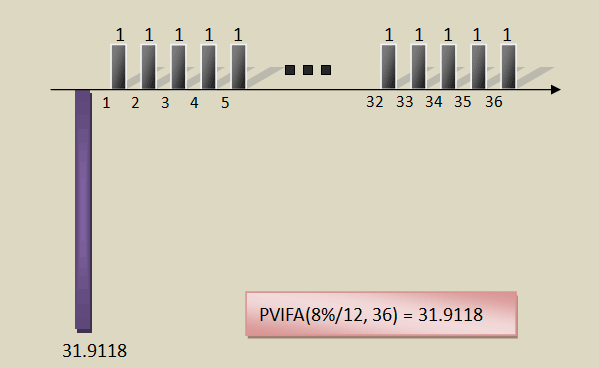﻿ 現值及未來值因子表

# 網路試算

 每期利率(r) % 期數(n) 期

## 未來值因子

FVIF(r,n) = (1+r)n

FVIF(2.5%, 5)是1元以2.5%利率5年後的本利和，所以100萬就是：
=1000000*FVIF(2.5%, 5) = 1000000*1.1314 = 1,131,400元

## 現值因子

PVIF(r,n) = 1 / (1+r)n

PVIF(2.3%, 20)是20年後一元之現值，所以只要乘上100就好：
=100*PVIF(2.3%, 20) = 100*0.6346 = 63.46元

## 年金未來值因子

FVIFA(r,n) = [(1+r)n-1] / r

=100000*FVIFA(10%, 10) = 100000*15.9374 = 1,593,740

## 年金現值因子

PVIFA(r,n) = [1-1/(1+r)n] / rPVIFA(8%/12, 36) = 31.9118，也可以解釋為銀行願意用31.9118元，來換取未來36期、每一期的1元的現金流量。也就是說每借31.9118元，以後36個月，每月都得還1元。那麼將貸款金額30萬除上31.9118，就是貸30萬每月之繳款金額了：
=300000/PVIFA(8%/12, 36) = 300000/31.9118 = 9,400.9元

# Excel檔案

﻿
﻿

■ 本網站內容儘可能精確完整，但不保證無誤。若做為投資依據，風險請自行斟酌 ，本網站不負賠償之責任。
■ 網站所有資料均為版權所有，非經書面允許請勿轉載或使用。